Question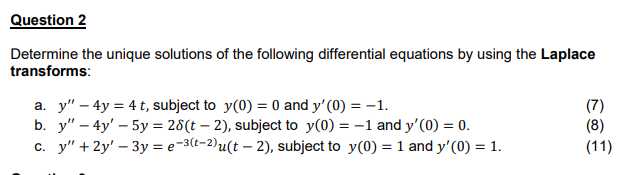We need at least 10 more requests to produce the answer.

0 / 10 have requested this problem solution

The more requests, the faster the answer.

All students who have requested the answer will be notified once they are available.

#### Earn Coins

Coins can be redeemed for fabulous gifts.

Similar Homework Help Questions
• ### Please solve using eigenvalues and vectors (Please do not use laplace transforms!) Please show all steps neatly and in detail, will rate quickly! 7. Find all the solutions to the following system of...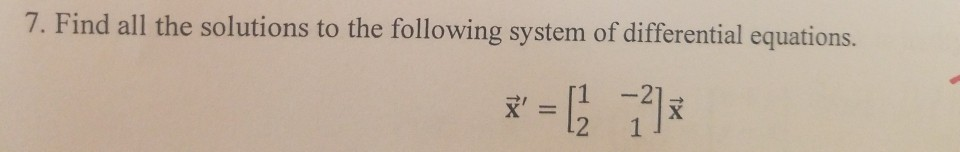Please solve using eigenvalues and vectors (Please do not use laplace transforms!) Please show all steps neatly and in detail, will rate quickly! 7. Find all the solutions to the following system of differential equations . ,-[1-21 7. Find all the solutions to the following system of differential equations . ,-[1-21

• ### Can you please show number #25, #27 (Please make work readable) 21. y" + 3y" +...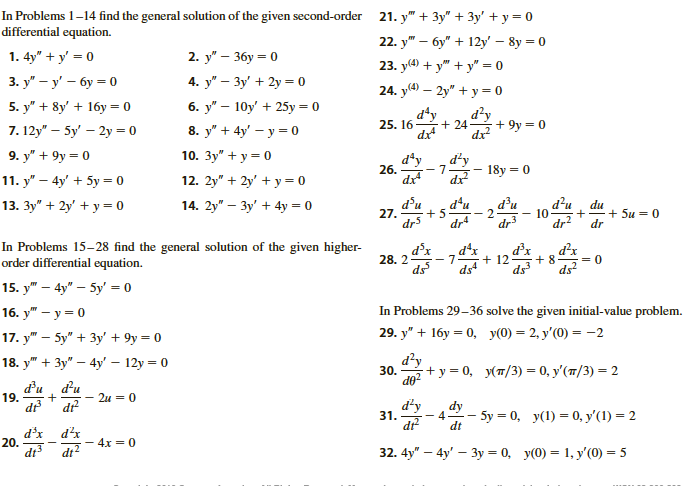Can you please show number #25, #27 (Please make work readable) 21. y" + 3y" + 3y' + y = 0 22. y" – 6y" + 12y' – 8y = 0 23. y(a) + y + y" =0 24. y(4) – 2y" +y=0 In Problems 1-14 find the general solution of the given second-order differential equation. 1. 4y" + y' = 0 2. y" – 36y = 0 3. y" - y' - 6y = 0 4. y" – 3y'...

• ### #3 please write solutions neatly 3. (17 points) Find the general solution of the linear differential...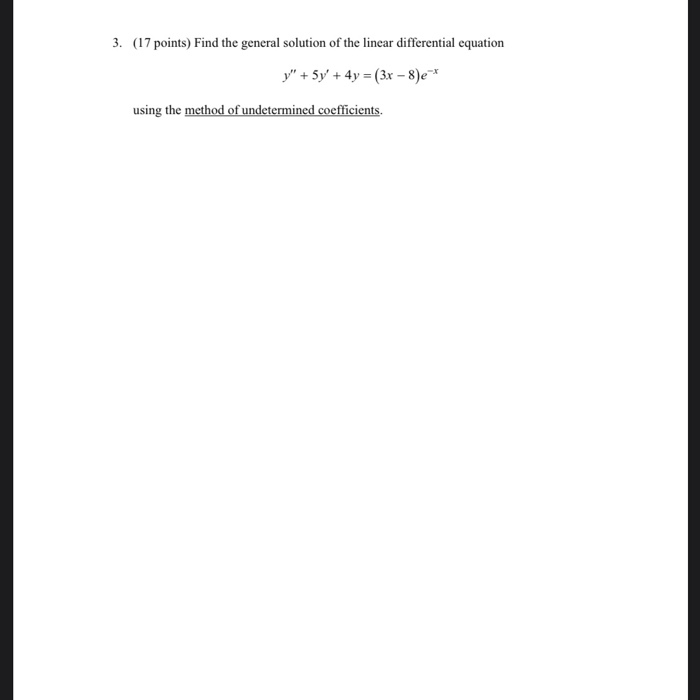#3 please write solutions neatly 3. (17 points) Find the general solution of the linear differential equation y" + 5y' + 4y = (3x - 8)e* using the method of undetermined coefficients.

• ### Please show solutions. Answer: 1. Find a general solution to the following differential equations: (a) y"...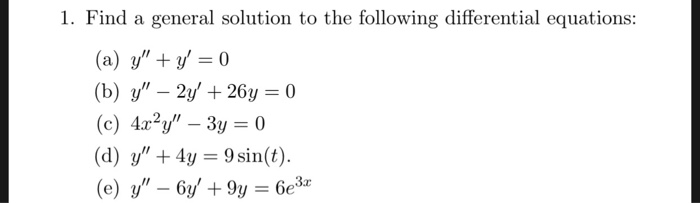Please show solutions. Answer: 1. Find a general solution to the following differential equations: (a) y" + y = 0 (b) y" – 2y' + 264 = 0 (c) 4x²y" – 3y = 0 (d) y" + 4y = 9 sin(t). (e) y" – 6y' + 9y = 6e3x 1. (a) y = ci + c2e- (b) y = cle' cos(5t) + czet sin(5t) (c) y = cit-1/2 + c2t3/2 (d) y = ci cos(2t) + c2 sin(2t) + 3...

• ### Please help solving all parts to this problem and show steps. (1 point) Use the Laplace...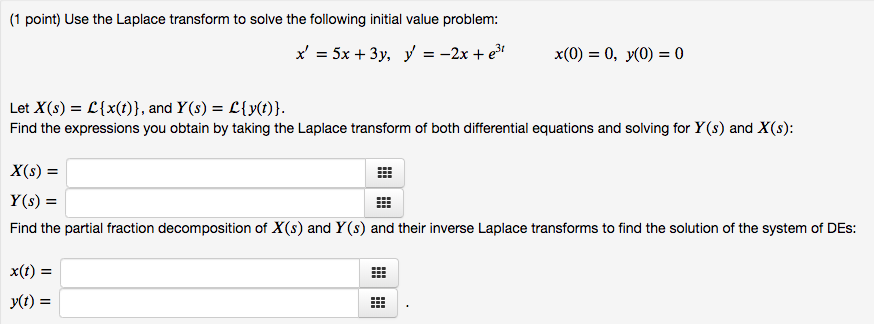Please help solving all parts to this problem and show steps. (1 point) Use the Laplace transform to solve the following initial value problem: x' = 5x + 3y, y = -2x +36 x(0) = 0, y0) = 0 Let X(s) = L{x(t)}, and Ys) = L{y(t)}. Find the expressions you obtain by taking the Laplace transform of both differential equations and solving for YS) and X (s): X(S) = Y(s) = Find the partial fraction decomposition of X(s) and...

• ### this is from differential equations ch8 section 2. please write clearly and show all steps Use...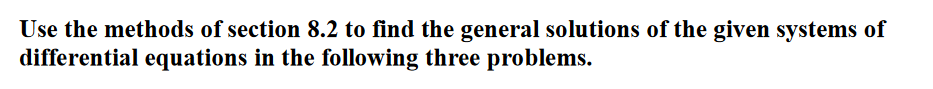this is from differential equations ch8 section 2. please write clearly and show all steps Use the methods of section 8.2 to find the general solutions of the given systems of differential equations in the following three problems. 2. dx dt 2x + y dy = -x + 4y dt

• ### please answer all questions and show all working 2. Solve the the following differential equations (you...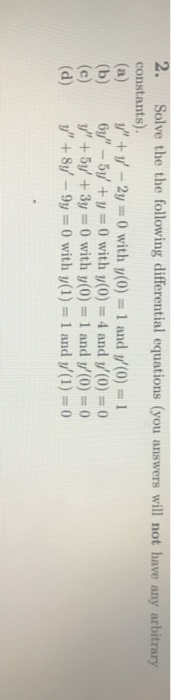please answer all questions and show all working 2. Solve the the following differential equations (you answers will not have any arbitrary constants) (a) y"+y' - 2y =0 with y(0) 1 and y(0) = 1 (b) 6y" - 5y + y =0 with y(0) = 4 and (0) = 0 (c) y" + 5y + 3y = 0 with y(0) = 1 and y(0) = 0 (d) y" + 8y' - 9y=0 with y(1) = 1 and y(1) = 0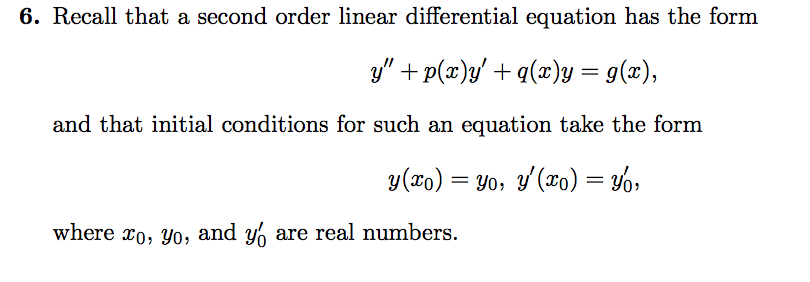please type your answer or write your answer neatly! 6. Recall that a second order linear differential equation has the form y" + p(x)y' + g(x)y = g(x), and that initial conditions for such an equation take the form y(20) = yo, y'(x0) = yo, where to, yo, and % are real numbers. (a) State carefully the fundamental existence and uniqueness theorem for such differ- ential equations. (Note: There are many equivalent ways to say the same thing. You need...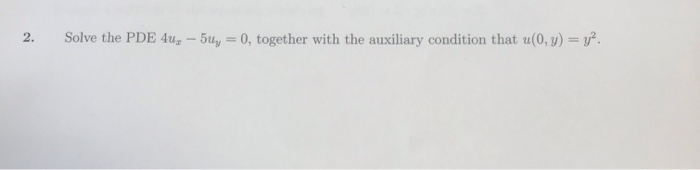Please answer the following questions please show all work and write your answers neatly and thoroughly please. Also please make sure your answers are correct. Thank you I appreciate it! 2. Solve the PDE 4ux - 5y = 0, together with the auxiliary condition that u(0,y) = y.

• ### please write neatly Solve the initial value problem using Laplace transforms. y = 2y + 12e-4,...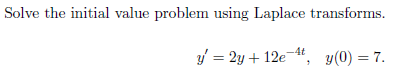please write neatly Solve the initial value problem using Laplace transforms. y = 2y + 12e-4, y(0) = 7.